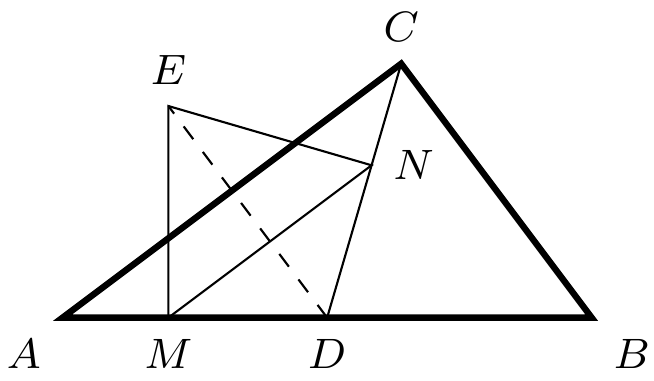###### back to index | new

Let $ABCDE$ be a pentagon inscribed in a circle such that $AB = CD = 3$, $BC = DE = 10$, and $AE= 14$. The sum of the lengths of all diagonals of $ABCDE$ is equal to $\frac{m}{n}$, where $m$ and $n$ are relatively prime positive integers. What is $m+n$ ?

A quadrilateral is inscribed in a circle of radius $200\sqrt{2}$. Three of the sides of this quadrilateral have length $200$. What is the length of the fourth side?

As shown, $\angle{ACB} = 90^\circ$, $AD=DB$, $DE=DC$, $EM\perp AB$, and $EN\perp CD$. Prove $$MN\cdot AB = AC\cdot CB$$What is the Ptolemy Theorem?